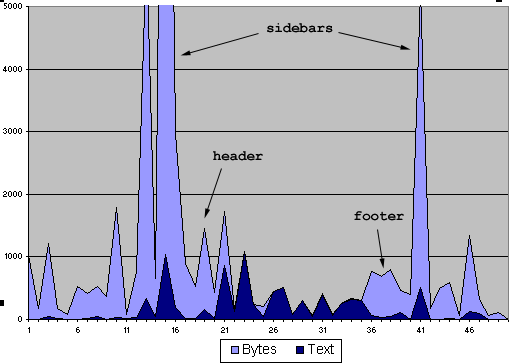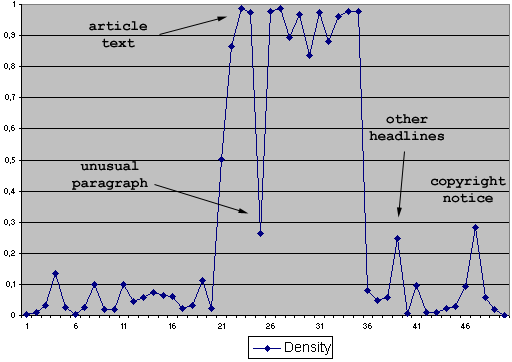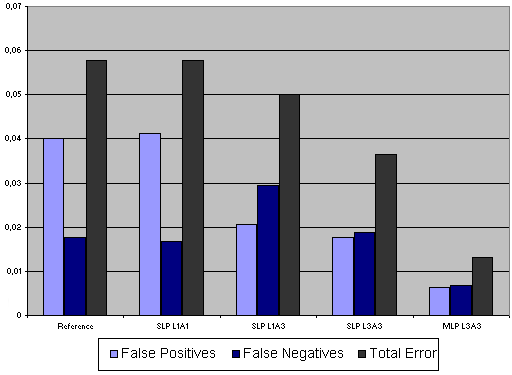# Python俱乐部

## 链接

python-files:html-body

# 从HTML文件中抽取正文的简单方案 zz

• 一、解析HTML代码并记下处理的字节数。
• 二、以行或段的形式保存解析输出的文本。
• 三、统计每一行文本相应的HTML代码的字节数
• 四、通过计算文本相对于字节数的比率来获取文本密度
• 五、最后用神经网络来决定这一行是不是正文的一部分。

## 转换HTML为文本

```def extract_text(html):
# Derive from formatter.AbstractWriter to store paragraphs.
writer = LineWriter()
# Default formatter sends commands to our writer.
formatter = AbstractFormatter(writer)
# Derive from htmllib.HTMLParser to track parsed bytes.
parser = TrackingParser(writer, formatter)
# Give the parser the raw HTML data.
parser.feed(html)
parser.close()
# Filter the paragraphs stored and output them.
return writer.output()```

TrackingParser覆盖了解析标签开始和结束时调用的回调函数，用以给缓冲对象传递当前解析的索引。通常你不得不这样，除非你使用不被推荐的方法——深入调用堆栈去获取执行帧。这个类看起来是这样的：

```class TrackingParser(htmllib.HTMLParser):
"""Try to keep accurate pointer of parsing location."""
def __init__(self, writer, *args):
htmllib.HTMLParser.__init__(self, *args)
self.writer = writer
def parse_starttag(self, i):
index = htmllib.HTMLParser.parse_starttag(self, i)
self.writer.index = index
return index
def parse_endtag(self, i):
self.writer.index = i
return htmllib.HTMLParser.parse_endtag(self, i)```

LinWriter的大部分工作都通过调用formatter来完成。如果你要改进或者修改程序，大部分时候其实就是在修改它。我们将在后面讲述怎么为它加上机器学习代码。但你也可以保持它的简单实现，仍然可以得到一个好结果。具体的代码如下：

```class Paragraph:
def __init__(self):
self.text = ''
self.bytes = 0
self.density = 0.0
class LineWriter(formatter.AbstractWriter):
def __init__(self, *args):
self.last_index = 0
self.lines = [Paragraph()]
formatter.AbstractWriter.__init__(self)
def send_flowing_data(self, data):
# Work out the length of this text chunk.
t = len(data)
# We've parsed more text, so increment index.
self.index += t
# Calculate the number of bytes since last time.
b = self.index - self.last_index
self.last_index = self.index
# Accumulate this information in current line.
l = self.lines[-1]
l.text += data
l.bytes += b
def send_paragraph(self, blankline):
"""Create a new paragraph if necessary."""
if self.lines[-1].text == '':
return
self.lines[-1].text += 'n' * (blankline+1)
self.lines[-1].bytes += 2 * (blankline+1)
self.lines.append(Writer.Paragraph())
def send_literal_data(self, data):
self.send_flowing_data(data)
def send_line_break(self):
self.send_paragraph(0)```

## 数据分析## 过滤文本行

```    def compute_density(self):
"""Calculate the density for each line, and the average."""
total = 0.0
for l in self.lines:
l.density = len(l.text) / float(l.bytes)
total += l.density
# Store for optional use by the neural network.
self.average = total / float(len(self.lines))
def output(self):
"""Return a string with the useless lines filtered out."""
self.compute_density()
output = StringIO.StringIO()
for l in self.lines:
# Check density against threshold.
# Custom filter extensions go here.
if l.density > 0.5:
output.write(l.text)
return output.getvalue()```

## 监督式机器学习• 当前行的密度
• 当前行的HTML字节数
• 当前行的输出文本长度
• 前一行的这三个值
• 后一行的这三个值

```from pyfann import fann, libfann
# This creates a new single-layer perceptron with 1 output and 3 inputs.
obj = libfann.fann_create_standard_array(2, (3, 1))
ann = fann.fann_class(obj)
# Load the data we described above.
ann.train_on_data(patterns, 1000, 1, 0.0)
# Then test it with different data.
for datin, datout in validation_data:
result = ann.run(datin)
print 'Got:', result, ' Expected:', datout```## 结论

python-files/html-body.txt · 最后更改: 2010/06/02 01:18 (外部编辑)

### 页面工具